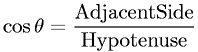Equations > Trigonometry > Trigonometric Definitions > Cosine Definition for a Right Triangle

### Cosine Definition for a Right TriangleLatex Code:

MathML Code:

 $\mathrm{cos}\theta =\frac\mathrm{Adjacent}\mathrm{Side}\mathrm{Hypotenuse}$

MathType 5.0: